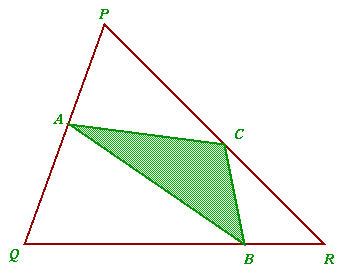You may also likeAreas and Ratios

Do you have enough information to work out the area of the shaded quadrilateral?Napoleon's Hat

Three equilateral triangles ABC, AYX and XZB are drawn with the point X a moveable point on AB. The points P, Q and R are the centres of the three triangles. What can you say about triangle PQR?Plane to See

P is the midpoint of an edge of a cube and Q divides another edge in the ratio 1 to 4. Find the ratio of the volumes of the two pieces of the cube cut by a plane through PQ and a vertex.

Halving the Triangle

Age 16 to 18 Challenge Level:
• Draw any triangle P, Q, R.
• Find points A, B and C, one on each side of the triangle (but not at the vertices), such that the area of triangle ABC is one quarter the area of triangle PQR.• Now find points such that the area of triangle ABC is one half the area of triangle PQR and a method that will give any fraction of the area between one quarter and one.
• How would you adapt your method to give fractions less than one quarter?